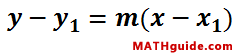The Point-Slope Formula
View the Lesson | MATHguide homepage Updated December 18th, 2016

Status: Waiting for your answers.Use the point-slope formula above to solve the problem below.

 Given: Two points, (10,3) and (-5,-6). Find: The equation of the line, in slope-intercept form [y = mx + b], that contains the given points.     y = -3/2 -3/5 -3/10 -1/2 -1/5 -1/10 1/2 1/5 1/10 3/2 3/5 3/10 x +